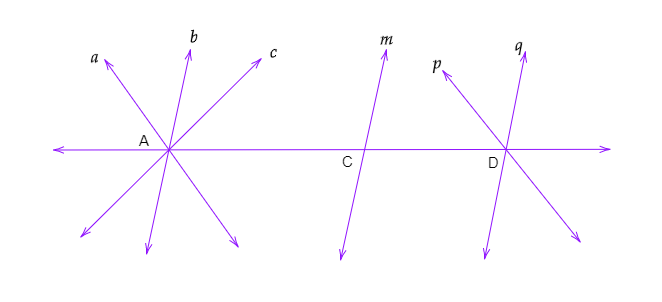"
">

# In the figure below , Name the parallel lines and the concurrent lines and points of concurrence in the figure."

Solution:Parallel lines-

In the given figure:

Lines $a$ and $p$ are parallel. $\Rightarrow a||p$

And lines $b$, $m$ and $q$ are parallel. $\Rightarrow b||m||q$.

Concurrent lines:-

Lines $a,\ b,\ c$ and line $AD$ are concurrent.

And $p$, $q$ and $AD$ are concurrent.

Point of concurrence:-

Point $A$ and $D$ are th4e points of concurrence.

Updated on: 10-Oct-2022

39 Views